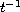Electron. J. Diff. Eqns., Vol. 2008(2008), No. 150, pp. 1-12.

### Dispersive estimates for a linear wave equation with electromagnetic potential Davide Catania

Abstract:
We consider radial solutions to the Cauchy problem for a linear wave equation with a small short-range electromagnetic potential (depending on space and time) and zero initial data. We present two dispersive estimates that provide, in particular, an optimal decay rate in timefor the solution. Also, we apply these estimates to obtain similar results for the linear massless Dirac equation perturbed by a potential.

Submitted December 12, 2006. Published October 29, 2008.
Math Subject Classifications: 35A08, 35L05, 35L15, 58J37, 58J45.
Key Words: Wave equation; electromagnetic potential; short-range; Dirac equation; dispersive estimate; decay estimate.

Show me the PDF file (239 KB), TEX file, and other files for this article.Davide Catania Dipartimento di Matematica, Facoltà di Ingegneria Università di Brescia, Via Valotti 9, 25133 Brescia, Italy email: catania@ing.unibs.it